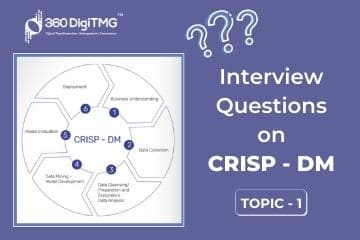Home / Blog / Interview Questions on Data Science / Moments of Business Decision

• September 30, 2020
• 6135
• 25### Meet the Author : Mr. Bharani Kumar

Bharani Kumar Depuru is a well known IT personality from Hyderabad. He is the Founder and Director of Innodatatics Pvt Ltd and 360DigiTMG. Bharani Kumar is an IIT and ISB alumni with more than 17 years of experience, he held prominent positions in the IT elites like HSBC, ITC Infotech, Infosys, and Deloitte. He is a prevalent IT consultant specializing in Industrial Revolution 4.0 implementation, Data Analytics practice setup, Artificial Intelligence, Big Data Analytics, Industrial IoT, Business Intelligence and Business Management. Bharani Kumar is also the chief trainer at 360DigiTMG with more than Ten years of experience and has been making the IT transition journey easy for his students. 360DigiTMG is at the forefront of delivering quality education, thereby bridging the gap between academia and industry.

1. ## What does “Central Tendency” mean to you?

The Mass/Concentration of the data is termed as the Central Tendency. It is a statistical measure that represents a value for the entire data.

2. ## Name the different measures of data used to evaluate the Central Tendency?

Mean, Median, and Mode are the three measures that are used for calculating the Central Tendency.

3. ## Define Mean?

The mean/average can be explained as the equal distribution of value to the total number of values. It is measured as the sum of the data points over the total number of values in the data.

4. ## What do you mean by Median?

Median is the middle-most value of all the data points after sorting.5. ## How can you define Mode?

The mode is known to be the most frequently occurring value, commonly used for working on categorical data.

6. ## Mention a disadvantage of Mean?

The biggest disadvantage is that the mean gets influenced by the outliers (also known as extreme values)

7. ## What do you mean by Dispersion?

Dispersion is the term used for the spread of data.

8. ## Mention all the measures used to analyze Dispersion?

The measures used for the analysis of Dispersion of data are Variance, Standard Deviation, and Range.9. ## Define the term Variance?

The average of the squared distance of each data point from the center (mean) is defined as Variance.

10. ## What are the disadvantages of Variance?

In Variance, we calculate the square for distance, but along with the distance, the unit also gets squared, so to get back units we use Standard Deviation.

11. ## What is Skewness?

Asymmetric of the distribution.

12. ## What are the thumb rules to interpret Skewness?

< +1 and > –1 a – Highly Skewed; Between –0.5 and –1 (or) +0.5 and +1 – Moderately Skewed; Between –0.5 and 0.5 – Approximately Symmetric.

13. ## What is Kurtosis?

Peakedness of the distribution. For Symmetric distributions, negative kurtosis implies a wider peak and thinner tails. For Symmetric distributions, positive kurtosis implies a narrow peak and wider tails.

14. ## Kurtosis can be calculated with which formula?

Kurtosis is a measure of the spread of the data from the centre. It is the 4th business moment which speaks about the shape of the distribution as being high peaked or wide peaked.

Kurtosis is calculated as the average distance of the data points from the centre.

∑_(i=1)^n [((x-μ)/σ)]4 - 315. ## What does Right Skewed mean?

Skewness is a measure that represents the mass of the data distributed towards the right side of the center. A histogram on the data will also enable us to understand the existence of extreme value in the dataset.

16. ## What does Left (or) Negative Skewed data mean?

Skewness is a measure that represents the mass of the data distributed towards the left side of the center. A histogram on the data will also enable us to understand the existence of extreme value in the dataset.

17. ## What is the measure of the Central tendency which changes with any single value change?

The Mean is calculated as the sum of the data points over the total number. The sum gets altered with a change in any single value in the data.

Median and Mode measures do not get affected by this change.

18. ## Variable X has a median of 50, the distribution of the data is positively skewed. Comment on the Mean of the Data?

The mean of the data is influenced by extreme values, whereas the median won't. Skewness in the data distribution is an effect of this extreme value. If the data is positively skewed that means the mean is pulled towards that positive side. Hence Mean is greater than the Median for such data. So we can conclude Mean > 50.

19. ## Can the measure of dispersion ‘Standard Deviation’ be negative?

The squared distance from the Mean to each data point is used in calculating the Standard Deviation. As we are squaring the values, we cannot get a negative value.

20. ## Does Standard Deviation get influenced by Outliers?

The distance of the data points from the center would be affected by outliers. Yes, the Standard Deviation would get effected.### Data Science Training Institutes in Other Locations